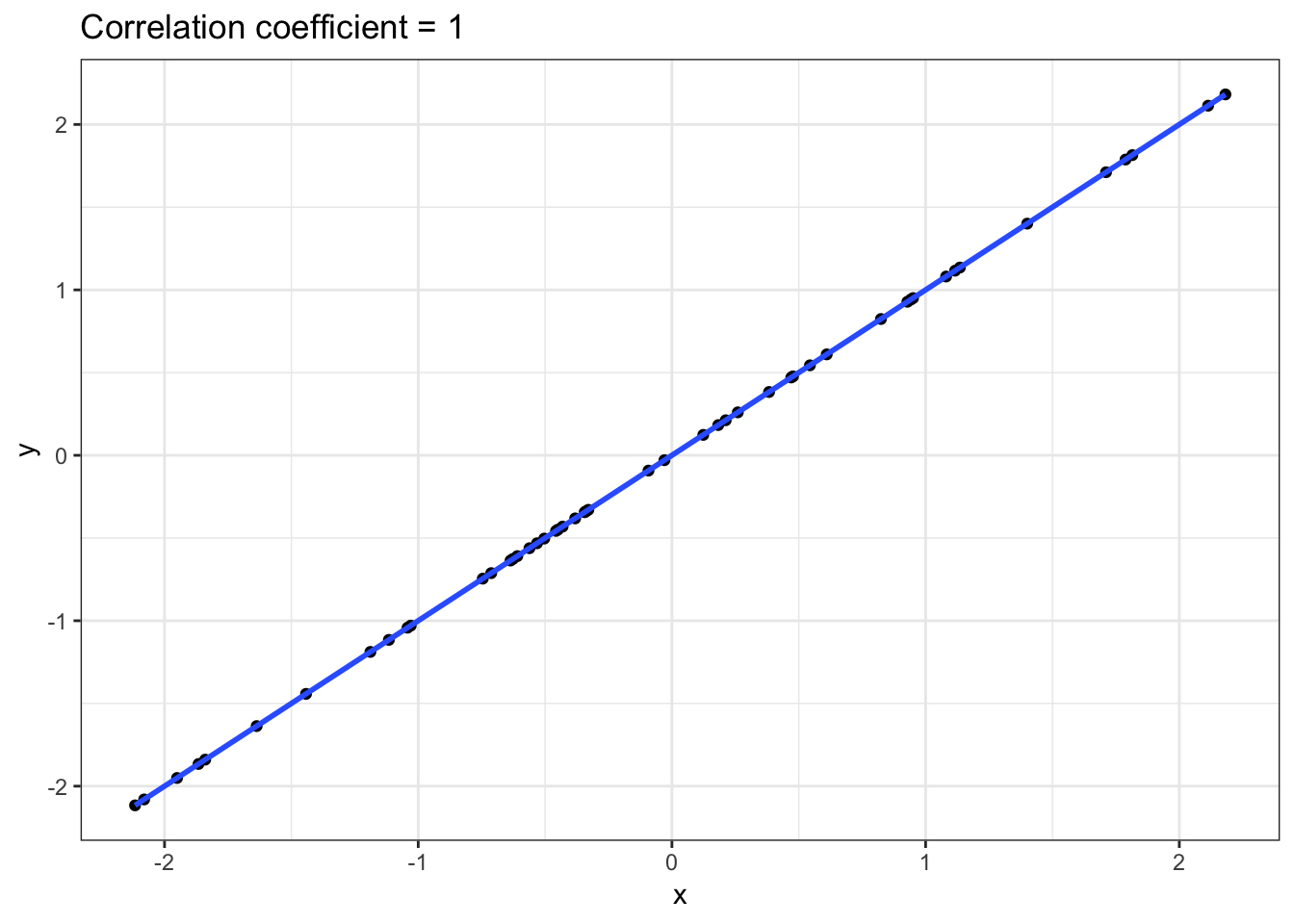## Introduction

One way to quantify the degree of agreement of two variables, such as drug response in the CCLE and GDSC studies, is to calculate the correlation between the pair. Correlation is commonly quantified as a value between -1 and 1, and measures the degree of association between the two variables. The higher the correlation value, the stronger the association. If two variables are exactly the same, then correlation is equal to 1. If two variables are unrelated, then correlation will be close to 0. What would a negative correlation mean?

When interpreting a correlation value, we consider how close the value is to 1 (or -1). There are no exact rules on calling a correlation “weak” or “strong”, and varies across scientific fields and applications. For the purposes of our analysis, we’ll consider values above 0.7 in magnitude as strong and below 0.3 as weak.

Note that there are several different types of correlations. For example, we might say that two variables are in agreement if they fall along a straight line when plotted against each other. Or, we might say they two are in agreement if an increase in one tends to be associated with an increase in the other (but not necessarily along a straight line). We’ll start by examining two different types for continuous variables:

1. Pearson’s correlation coefficient: measures the degree of linear between variables,

2. Spearman’s correlation coefficient: measures the agreement of the rankings between variables.

We’ll also briefly introduce a third measure of correlation:

1. Matthews’ correlation coefficient: measures the degree of agreement between categorical variables.

## Setup Workspace

We start by loading the tidyverse family of packages and specifying a default plotting theme for our `ggplot` graphics.

``library(tidyverse)``
``````## Registered S3 methods overwritten by 'ggplot2':
##   method         from
##   [.quosures     rlang
##   c.quosures     rlang
##   print.quosures rlang``````
``## ── Attaching packages ────────────────────────────────── tidyverse 1.2.1 ──``
``````## ✔ ggplot2 3.1.1     ✔ purrr   0.3.2
## ✔ tibble  2.1.2     ✔ dplyr   0.8.1
## ✔ tidyr   0.8.3     ✔ stringr 1.4.0
## ✔ readr   1.3.1     ✔ forcats 0.4.0``````
``````## ── Conflicts ───────────────────────────────────── tidyverse_conflicts() ──
``theme_set(theme_bw())``

## Pearson Correlation

Here are some example scatterplots and their resulting correlation coefficients using the Pearson measure of linear association.

``````# set seed for reproducibility
set.seed(738)

# Perfect correlation
x <- rnorm(50)
perfect <- data.frame(x=x, y=x)
cor.coef <- round(cor(perfect\$x, perfect\$y),2)
ggplot(data=perfect, aes(x=x,y=y)) +
geom_point() +
ggtitle(paste0("Correlation coefficient = ", cor.coef)) +
geom_smooth(method='lm', se=FALSE)````````````# Strong correlation
x <- rnorm(50,0,2)
strong <- data.frame(x=x, y=x+rnorm(50,0,0.75))
cor.coef <- round(cor(strong\$x, strong\$y),2)
ggplot(data=strong, aes(x=x,y=y)) +
geom_point() +
ggtitle(paste0("Correlation coefficient = ", cor.coef))+
geom_smooth(method='lm', se=FALSE)``````